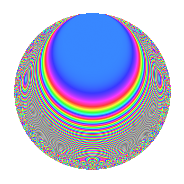# Properties

 Label 224.3.nLevel 224 Weight 3 Character orbit n Rep. character $$\chi_{224}(17,\cdot)$$ Character field $$\Q(\zeta_{6})$$ Dimension 28 Newform subspaces 1 Sturm bound 96 Trace bound 0

# Related objects

## Defining parameters

 Level: $$N$$ $$=$$ $$224 = 2^{5} \cdot 7$$ Weight: $$k$$ $$=$$ $$3$$ Character orbit: $$[\chi]$$ $$=$$ 224.n (of order $$6$$ and degree $$2$$) Character conductor: $$\operatorname{cond}(\chi)$$ $$=$$ $$56$$ Character field: $$\Q(\zeta_{6})$$ Newform subspaces: $$1$$ Sturm bound: $$96$$ Trace bound: $$0$$

## Dimensions

The following table gives the dimensions of various subspaces of $$M_{3}(224, [\chi])$$.

Total New Old
Modular forms 144 36 108
Cusp forms 112 28 84
Eisenstein series 32 8 24

## Trace form

 $$28q + 4q^{7} - 32q^{9} + O(q^{10})$$ $$28q + 4q^{7} - 32q^{9} - 28q^{15} - 6q^{17} - 30q^{23} - 32q^{25} + 6q^{31} - 6q^{33} + 20q^{39} + 294q^{47} - 20q^{49} + 124q^{57} - 432q^{63} - 52q^{65} + 136q^{71} + 234q^{73} + 162q^{79} - 18q^{81} - 48q^{87} - 150q^{89} - 290q^{95} + O(q^{100})$$

## Decomposition of $$S_{3}^{\mathrm{new}}(224, [\chi])$$ into newform subspaces

Label Dim. $$A$$ Field CM Traces $q$-expansion
$$a_2$$ $$a_3$$ $$a_5$$ $$a_7$$
224.3.n.a $$28$$ $$6.104$$ None $$0$$ $$0$$ $$0$$ $$4$$

## Decomposition of $$S_{3}^{\mathrm{old}}(224, [\chi])$$ into lower level spaces

$$S_{3}^{\mathrm{old}}(224, [\chi]) \cong$$ $$S_{3}^{\mathrm{new}}(56, [\chi])$$$$^{\oplus 3}$$

## Hecke characteristic polynomials

There are no characteristic polynomials of Hecke operators in the database